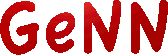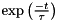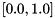GeNN  3.2.0 GPU enhanced Neuronal Networks (GeNN)
Neuron models

There is a number of predefined models which can be used with the NNmodel::addNeuronGroup function:

# Defining your own neuron type

In order to define a new neuron type for use in a GeNN application, it is necessary to define a new class derived from NeuronModels::Base. For convenience the methods this class should implement can be implemented using macros:

• DECLARE_MODEL(TYPE, NUM_PARAMS, NUM_VARS): declared the boilerplate code required for the model e.g. the correct specialisations of NewModels::ValueBase used to wrap the neuron model parameters and values.
• SET_SIM_CODE(SIM_CODE): where SIM_CODE contains the code for executing the integration of the model for one time stepWithin this code string, variables need to be referred to by $(NAME), where NAME is the name of the variable as defined in the vector varNames. The code may refer to the predefined primitives DT for the time step size and for the total incoming synaptic current. It can also refer to a unique ID (within the population) using . • SET_THRESHOLD_CONDITION_CODE(THRESHOLD_CONDITION_CODE) defines the condition for true spike detection. • SET_PARAM_NAMES() defines the names of the model parameters. If defined as NAME here, they can then be referenced as$(NAME) in the code string. The length of this list should match the NUM_PARAM specified in DECLARE_MODEL. Parameters are assumed to be always of type double.
• SET_VARS() defines the names and type strings (e.g. "float", "double", etc) of the neuron state variables. The type string "scalar" can be used for variables which should be implemented using the precision set globally for the model with NNmodel::setPrecision. The variables defined here as NAME can then be used in the syntax $(NAME) in the code string. For example, using these macros, we can define a leaky integratorsolved using Euler's method: class LeakyIntegrator : public NeuronModels::Base { public: DECLARE_MODEL(LeakyIntegrator, 1, 1); SET_SIM_CODE("$(V)+= (-$(V)+$(Isyn))*(DT/$(tau));"); SET_PARAM_NAMES({"tau"}); SET_VARS({{"V", "scalar"}}); }; Additionally "dependent parameters" can be defined. Dependent parameters are a mechanism for enhanced efficiency when running neuron models. If parameters with model-side meaning, such as time constants or conductances always appear in a certain combination in the model, then it is more efficient to pre-compute this combination and define it as a dependent parameter. For example, because the equation defining the previous leaky integrator example has an algebraic solution, it can be more accurately solved as follows - using a derived parameter to calculate: class LeakyIntegrator2 : public NeuronModels::Base { public: DECLARE_MODEL(LeakyIntegrator2, 1, 1); SET_SIM_CODE("$(V) = $(Isyn) -$(ExpTC)*($(Isyn) -$(V));");
SET_PARAM_NAMES({"tau"});
SET_VARS({{"V", "scalar"}});
{"ExpTC", [](const vector<double> &pars, double dt){ return std::exp(-dt / pars); }}});
};

GeNN provides several additional features that might be useful when defining more complex neuron models.

## Support code

Support code enables a code block to be defined that contains supporting code that will be utilized in multiple pieces of user code. Typically, these are functions that are needed in the sim code or threshold condition code. If possible, these should be defined as __host__ __device__ functions so that both GPU and CPU versions of GeNN code have an appropriate support code function available. The support code is protected with a namespace so that it is exclusively available for the neuron population whose neurons define it. Support code is added to a model using the SET_SUPPORT_CODE() macro, for example:

SET_SUPPORT_CODE("__device__ __host__ scalar mysin(float x){ return sin(x); }");

## Extra global parameters

Extra global parameters are parameters common to all neurons in the population. However, unlike the standard neuron parameters, they can be varied at runtime meaning they could, for example, be used to provide a global reward signal. These parameters are defined by using the SET_EXTRA_GLOBAL_PARAMS() macro to specify a list of variable names and type strings (like the SET_VARS() macro). For example:

SET_EXTRA_GLOBAL_PARAMS({{"R", "float"}});

These variables are available to all neurons in the population. They can also be used in synaptic code snippets; in this case it need to be addressed with a _pre or _post postfix.

For example, if the model with the "R" parameter was used for the pre-synaptic neuron population, the weight update model of a synapse population could have simulation code like:

SET_SIM_CODE("$(x)=$(x)+$(R_pre);"); where we have assumed that the weight update model has a variable x and our synapse type will only be used in conjunction with pre-synaptic neuron populations that do have the extra global parameter R. If the pre-synaptic population does not have the required variable/parameter, GeNN will fail when compiling the kernels. ## Additional input variables Normally, neuron models receive the linear sum of the inputs coming from all of their synaptic inputs through the$(inSyn) variable. However neuron models can define additional input variables - allowing input from different synaptic inputs to be combined non-linearly. For example, if we wanted our leaky integrator to operate on the the product of two input currents, it could be defined as follows:

SET_SIM_CODE("const scalar input = $(Isyn) *$(Isyn2);\n"
"$(V) = input -$(ExpTC)*(input - $(V));"); Where the SET_ADDITIONAL_INPUT_VARS() macro defines the name, type and its initial value before postsynaptic inputs are applyed (see section Postsynaptic integration methods for more details). ## Random number generation Many neuron models have probabilistic terms, for example a source of noise or a probabilistic spiking mechanism. In GeNN this can be implemented by using the following functions in blocks of model code: • $(gennrand_uniform) returns a number drawn uniformly from the interval• $(gennrand_normal) returns a number drawn from a normal distribution with a mean of 0 and a standard deviation of 1. • $(gennrand_exponential) returns a number drawn from an exponential distribution with.
• \$(gennrand_log_normal, MEAN, STDDEV) returns a number drawn from a log-normal distribution with the specified mean and standard deviation.